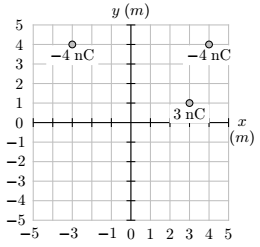# Problem: Let: V = 0 at infinity. Three charges are arranged in the (x, y) plane (as shown in the figure below, where the scale is in meters). Find the electric potential Vo at the origin [coordinates (0 m, 0 m)].

###### FREE Expert Solution
99% (234 ratings)
###### Problem Details

Let: V = 0 at infinity. Three charges are arranged in the (x, y) plane (as shown in the figure below, where the scale is in meters). Find the electric potential Vo at the origin [coordinates (0 m, 0 m)].